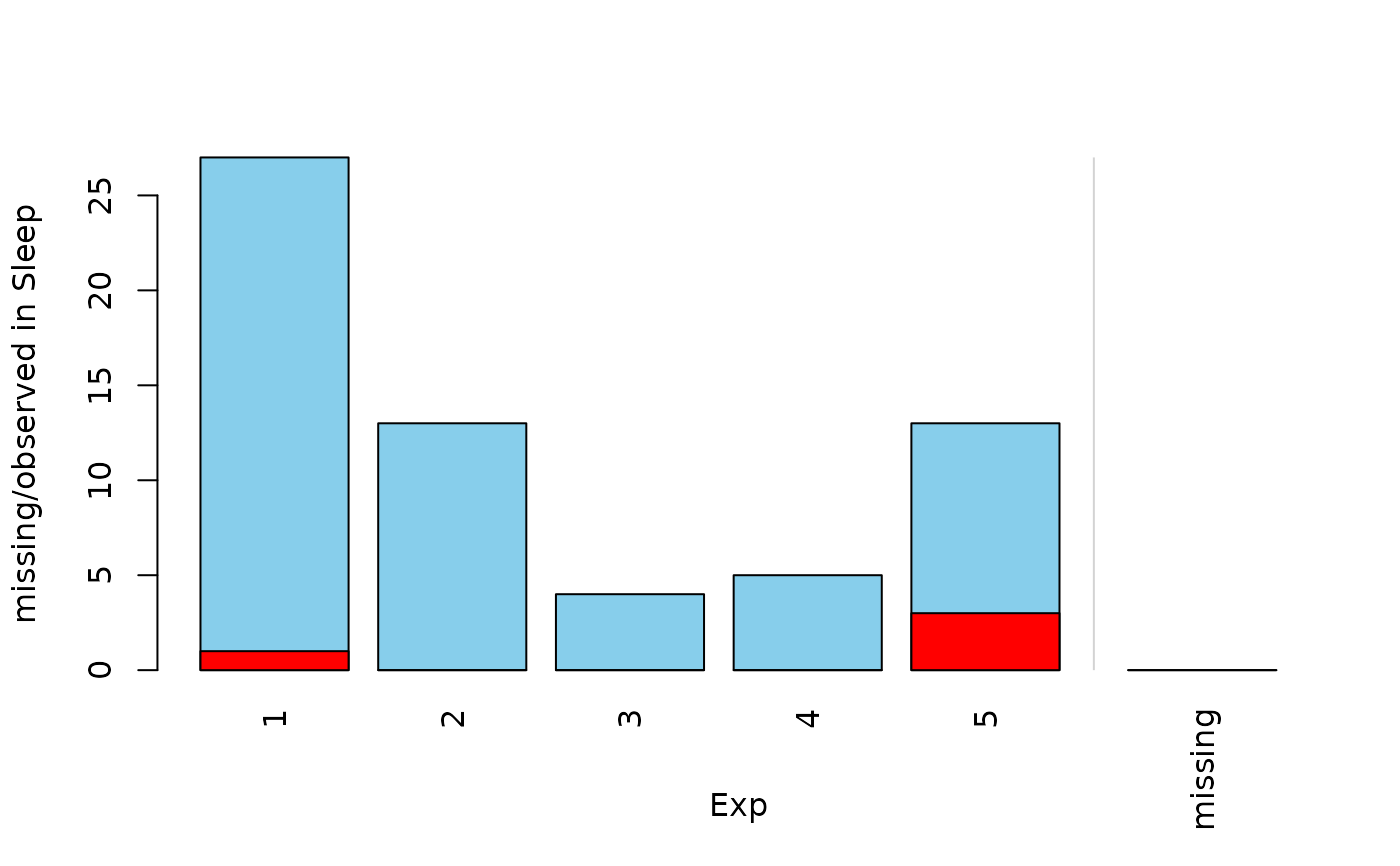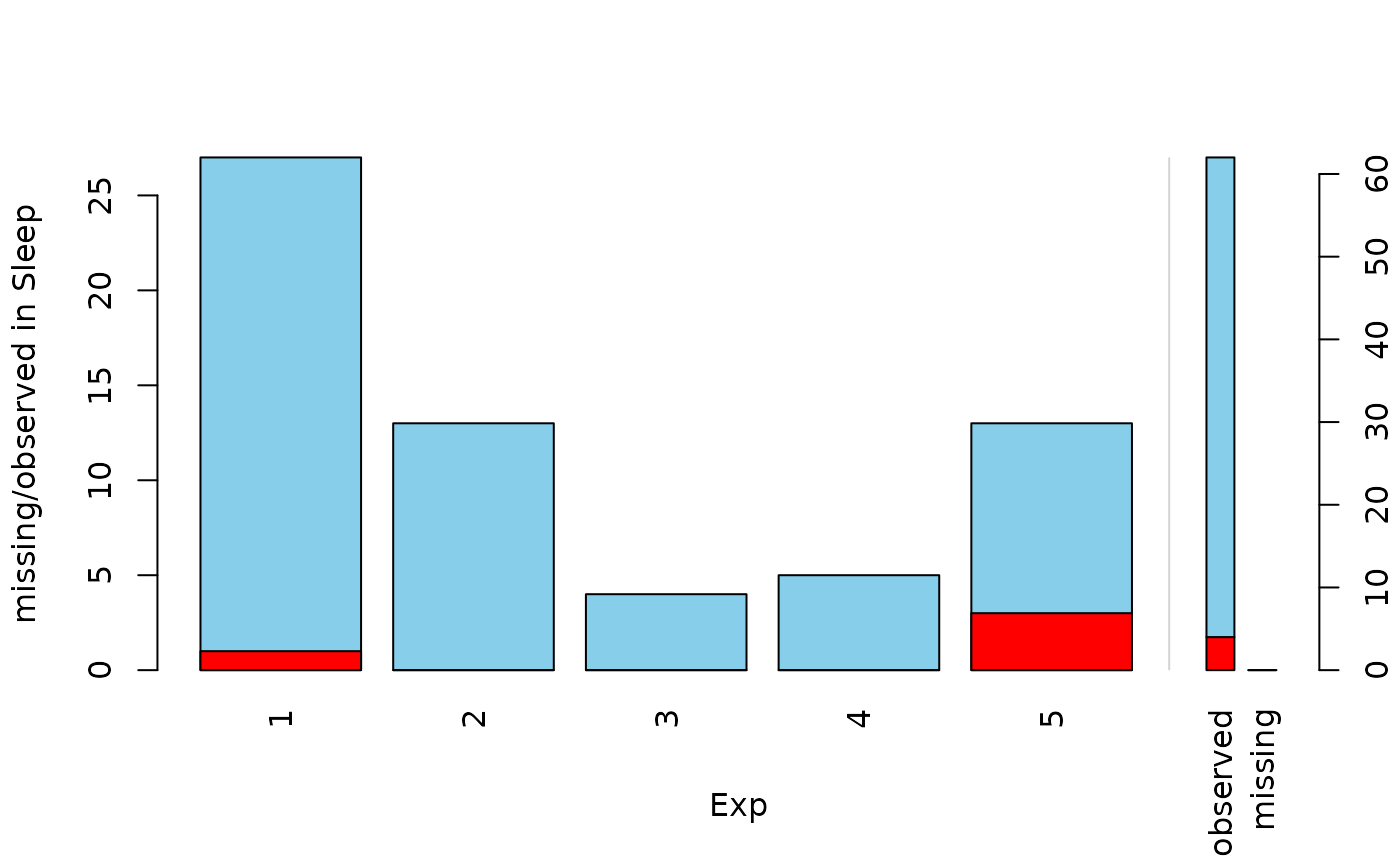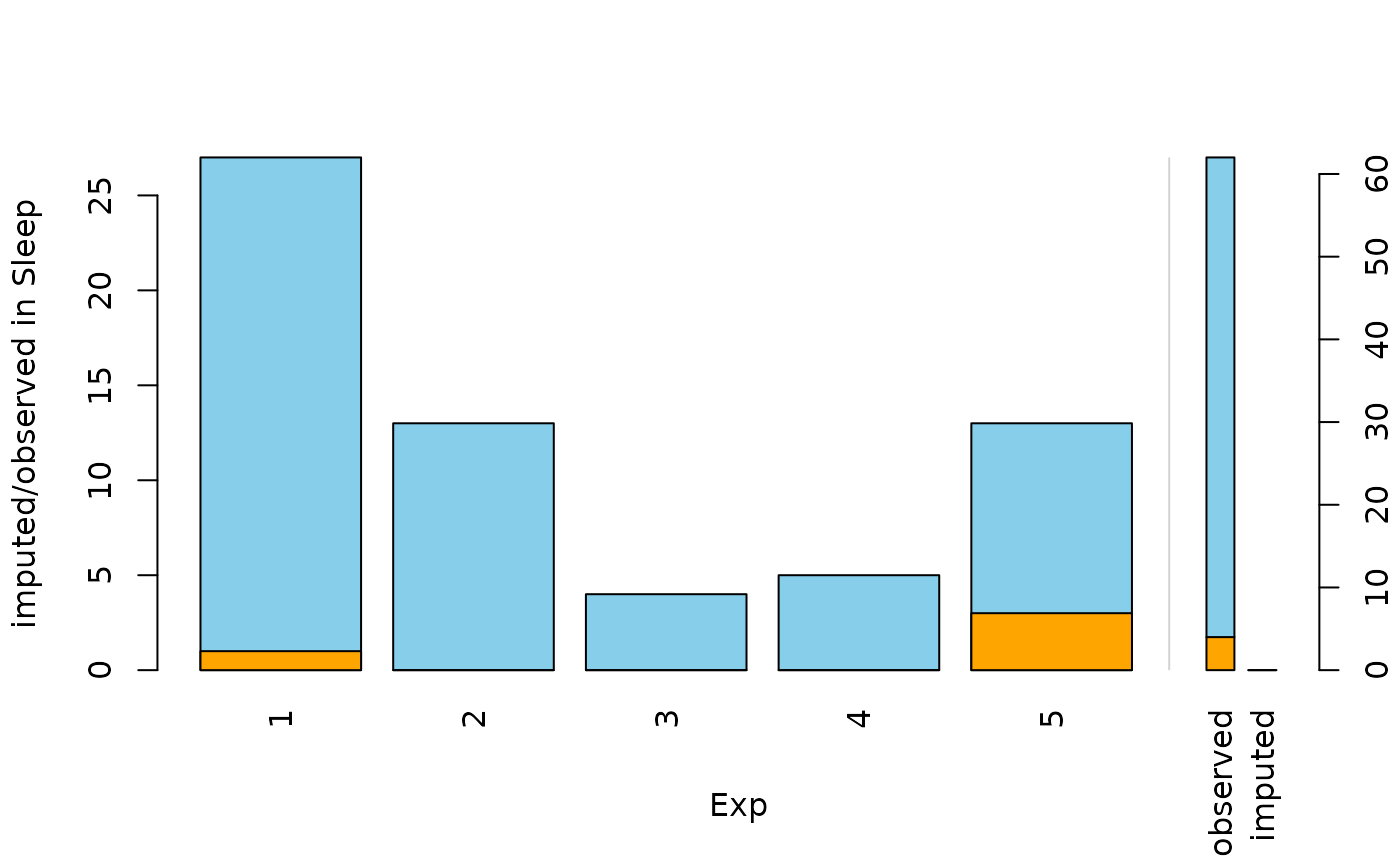Barplot with highlighting of missing/imputed values in other variables by splitting each bar into two parts. Additionally, information about missing/imputed values in the variable of interest is shown on the right hand side.

barMiss(
x,
delimiter = NULL,
pos = 1,
selection = c("any", "all"),
col = c("skyblue", "red", "skyblue4", "red4", "orange", "orange4"),
border = NULL,
main = NULL,
sub = NULL,
xlab = NULL,
ylab = NULL,
axes = TRUE,
labels = axes,
only.miss = TRUE,
miss.labels = axes,
interactive = TRUE,
...
)

## Arguments

x

a vector, matrix or data.frame.

delimiter

a character-vector to distinguish between variables and imputation-indices for imputed variables (therefore, x needs to have colnames()). If given, it is used to determine the corresponding imputation-index for any imputed variable (a logical-vector indicating which values of the variable have been imputed). If such imputation-indices are found, they are used for highlighting and the colors are adjusted according to the given colors for imputed variables (see col).

pos

a numeric value giving the index of the variable of interest. Additional variables in x are used for highlighting.

selection

the selection method for highlighting missing/imputed values in multiple additional variables. Possible values are "any" (highlighting of missing/imputed values in any of the additional variables) and "all" (highlighting of missing/imputed values in all of the additional variables).

col

a vector of length six giving the colors to be used. If only one color is supplied, the bars are transparent and the supplied color is used for highlighting missing/imputed values. Else if two colors are supplied, they are recycled.

border

the color to be used for the border of the bars. Use border=NA to omit borders.

main, sub

main and sub title.

xlab, ylab

axis labels.

axes

a logical indicating whether axes should be drawn on the plot.

labels

either a logical indicating whether labels should be plotted below each bar, or a character vector giving the labels.

only.miss

logical; if TRUE, the missing/imputed values in the variable of interest are visualized by a single bar. Otherwise, a small barplot is drawn on the right hand side (see ‘Details’).

miss.labels

either a logical indicating whether label(s) should be plotted below the bar(s) on the right hand side, or a character string or vector giving the label(s) (see ‘Details’).

interactive

a logical indicating whether variables can be switched interactively (see ‘Details’).

...

further graphical parameters to be passed to graphics::title() and graphics::axis().

## Value

a numeric vector giving the coordinates of the midpoints of the bars.

## Details

If more than one variable is supplied, the bars for the variable of interest are split according to missingness/number of imputed missings in the additional variables.

If only.miss=TRUE, the missing/imputed values in the variable of interest are visualized by one bar on the right hand side. If additional variables are supplied, this bar is again split into two parts according to missingness/number of imputed missings in the additional variables.

Otherwise, a small barplot consisting of two bars is drawn on the right hand side. The first bar corresponds to observed values in the variable of interest and the second bar to missing/imputed values. Since these two bars are not on the same scale as the main barplot, a second y-axis is plotted on the right (if axes=TRUE). Each of the two bars are again split into two parts according to missingness/number of imputed missings in the additional variables. Note that this display does not make sense if only one variable is supplied, therefore only.miss is ignored in that case.

If interactive=TRUE, clicking in the left margin of the plot results in switching to the previous variable and clicking in the right margin results in switching to the next variable. Clicking anywhere else on the graphics device quits the interactive session. When switching to a continuous variable, a histogram is plotted rather than a barplot.

## Note

Some of the argument names and positions have changed with version 1.3 due to extended functionality and for more consistency with other plot functions in VIM. For back compatibility, the arguments axisnames, names.arg and names.miss can still be supplied to ...{} and are handled correctly. Nevertheless, they are deprecated and no longer documented. Use labels and miss.labels instead.

spineMiss(), histMiss()

Other plotting functions: aggr(), histMiss(), marginmatrix(), marginplot(), matrixplot(), mosaicMiss(), pairsVIM(), parcoordMiss(), pbox(), scattJitt(), scattMiss(), scattmatrixMiss(), spineMiss()

## Author

Andreas Alfons, modifications to show imputed values by Bernd Prantner

## Examples


data(sleep, package = "VIM")
## for missing values
x <- sleep[, c("Exp", "Sleep")]
barMiss(x)barMiss(x, only.miss = FALSE)## for imputed values
x_IMPUTED  <- kNN(sleep[, c("Exp", "Sleep")])
barMiss(x_IMPUTED, delimiter = "_imp")barMiss(x_IMPUTED, delimiter = "_imp", only.miss = FALSE)